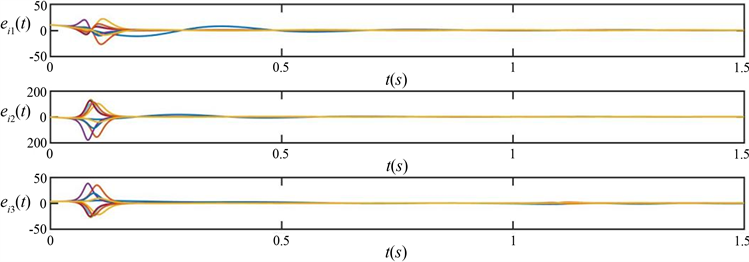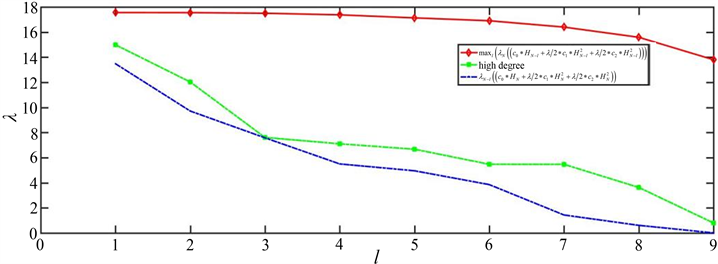#### 期刊菜单

Optimal Pinning Control Synchronization of Node Groups for Multi-Edges Complex Networks

Abstract: Aiming at pinning synchronization control node’s selection in multi-edges complex networks, a strategy based on graph theory to optimize the selection of pinning node groups is proposed. According to the edges’ properties that is different in the network, based on the network splitting’s idea, the network is split by introducing delay. According to Lyapunov stability theory, using adaptive pinning controller, graph theory and matrix analysis’s knowledge, a scheme that can optimize pinning node groups’ selection in multi-edges complex networks is obtained: When the pinning nodes’ number is fixed, the maximum eigenvalue of the sub matrix obtained by deleting the rows and columns corresponding to the controlled nodes from the symmetric Laplacian matrix of the network is used to measure the pinning scheme’s effectiveness. That is, when the dynamic system and nodes’ coupling strength in the network are given, the larger the maximum eigenvalue, the better the effectiveness of the pinning scheme. At the same time, the spectral characteristics of the maximum eigenvalue are analyzed, which provides a theoretical support for the optimization of the pinning scheme.

1. 引言

2. 模型准备及描述

$\stackrel{˙}{x}\left(t\right)=f\left({x}_{i}\left(t\right)\right)-c\underset{j=1}{\overset{N}{\sum }}{P}_{ij}H{x}_{j}\text{}\left(i=1,2,\cdots ,N\right)$ (1)

$\begin{array}{c}{\stackrel{˙}{x}}_{i}\left(t\right)=f\left({x}_{i}\left(t\right),t\right)-{c}_{0}\underset{j=1}{\overset{N}{\sum }}{p}_{\left(0\right)}{}_{ij}H{x}_{j}\left(t\right)-{c}_{1}\underset{j=1}{\overset{N}{\sum }}{p}_{\left(1\right)}{}_{ij}H{x}_{j}\left(t-{\tau }_{1}\right)-{c}_{2}\underset{j=1}{\overset{N}{\sum }}{p}_{\left(2\right)}{}_{ij}H{x}_{j}\left(t-{\tau }_{2}\right)\\ \text{\hspace{0.17em}}\text{ }\text{ }+\cdots -{c}_{k}\underset{j=1}{\overset{N}{\sum }}{p}_{\left(k\right)}{}_{ij}H{x}_{j}\left(t-{\tau }_{k}\right)\end{array}$ (2)

${P}_{N\left(r\right)}={\left[{p}_{\left(r\right)}{}_{ij}\right]}_{N×N}$ 表示第r个子网络的外部耦合矩阵，此处我们将其以拉普拉斯矩阵的形式表现出来， $r=0,1,\cdots ,k$ ，可以用来展示网络间的拓扑结构，并且满足耗散耦合条件，其中矩阵 ${P}_{N}={\left[{p}_{ij}\right]}_{N×N}$ 定义如

$\underset{t\to \infty }{\mathrm{lim}}‖{x}_{i}\left(t\right)-s\left(t\right)‖=0,\text{}i=1,2,\cdots ,N.$ (3)

$\stackrel{˙}{s}\left(t\right)=f\left(s\left(t\right),t\right)$ (4)

${e}_{i}\left(t\right)={x}_{i}\left(t\right)-s\left(t\right),\text{}i=1,2,\cdots ,N.$ (5)

${\stackrel{˙}{x}}_{i}\left(t\right)=f\left({x}_{i}\left(t\right),t\right)-{c}_{0}\underset{j=1}{\overset{N}{\sum }}{p}_{\left(0\right)}{}_{ij}H{x}_{j}\left(t\right)-\underset{r=1}{\overset{k}{\sum }}{c}_{r}\underset{j=1}{\overset{N}{\sum }}{p}_{\left(r\right)}{}_{ij}H{x}_{j}\left(t-{\tau }_{r}\right)+{u}_{i}\left(t\right),\text{}i=1,2,\cdots ,l$

${\stackrel{˙}{x}}_{i}\left(t\right)=f\left({x}_{i}\left(t\right),t\right)-{c}_{0}\underset{j=1}{\overset{N}{\sum }}{p}_{\left(0\right)}{}_{ij}H{x}_{j}\left(t\right)-\underset{r=1}{\overset{k}{\sum }}{c}_{r}\underset{j=1}{\overset{N}{\sum }}{p}_{\left(r\right)}{}_{ij}H{x}_{j}\left(t-{\tau }_{r}\right),\text{}i=l+1,l+2,\cdots ,N.$ (6)

${u}_{i}\left(t\right)=\left\{\begin{array}{l}-{d}_{i}\left(t\right)H{e}_{i}\left(t\right),\text{}1\le i\le l\\ 0,\text{}l+1\le i\le N\end{array}$ (7)

${\stackrel{˙}{e}}_{i}\left(t\right)=f\left({x}_{i}\left(t\right),t\right)-f\left(s\left(t\right),t\right)-{c}_{0}\underset{j=1}{\overset{N}{\sum }}{p}_{\left(0\right)}{}_{ij}H{e}_{j}\left(t\right)-\underset{r=1}{\overset{k}{\sum }}{c}_{r}\underset{j=1}{\overset{N}{\sum }}{p}_{\left(r\right)}{}_{ij}H{e}_{j}\left(t-{\tau }_{r}\right)+{u}_{i}\left( t \right)$

${\stackrel{˙}{e}}_{i}\left(t\right)=f\left({x}_{i}\left(t\right),t\right)-f\left(s\left(t\right),t\right)-{c}_{0}\underset{j=1}{\overset{N}{\sum }}{p}_{\left(0\right)}{}_{ij}H{e}_{j}\left(t\right)-\underset{r=1}{\overset{k}{\sum }}{c}_{r}\underset{j=1}{\overset{N}{\sum }}{p}_{\left(r\right)}{}_{ij}H{e}_{j}\left(t-{\tau }_{r}\right)$ (8)

${\left(x-y\right)}^{\text{T}}\left(f\left(x\right)-f\left(y\right)\right)\le \vartheta {\left(x-y\right)}^{\text{T}}H\left(x-y\right),\text{}\forall x,y\in {R}^{m}$ (9)

${\lambda }_{1}\left(B\right)\le \underset{1\le i\le N}{\mathrm{min}}\left[{b}_{ii}\right]\le \underset{1\le i\le N}{\mathrm{max}}\left[{b}_{ii}\right]\le {\lambda }_{N}\left(B\right)$ (10)

${\lambda }_{1}\left(B\right)$${\lambda }_{N}\left(B\right)$ 分别表示矩阵B的最小特征值和最大特征值。

$\left(\begin{array}{cc}U\left(x\right)& S\left(x\right)\\ {S}^{\text{T}}\left(x\right)& W\left(x\right)\end{array}\right)<0,$ (11)

$\left(1\right)\text{\hspace{0.17em}}U\left(x\right)<0,\text{}W\left(x\right)-S{\left(x\right)}^{\text{T}}U{\left(x\right)}^{-1}S\left(x\right)<0,$

$\left(2\right)\text{\hspace{0.17em}}W\left(x\right)<0,\text{}U\left(x\right)-S\left(x\right)W{\left(x\right)}^{-1}S{\left(x\right)}^{\text{T}}<0.$ (12)

${\lambda }_{1}\le {\mu }_{1}\le {\lambda }_{2}\le {\mu }_{2}\le \cdots \le {\lambda }_{N-1}\le {\mu }_{N-1}\le {\lambda }_{N}$ (13)

$2{x}^{\text{T}}y\le {x}^{\text{T}}Wx+{y}^{\text{T}}{W}^{-1}y$ (14)

$\left(1\right)\text{\hspace{0.17em}}{\left(U\otimes Y\right)}^{\text{T}}={U}^{\text{T}}\otimes {Y}^{\text{T}};$

$\left(2\right)\text{\hspace{0.17em}}\left(U\otimes Y\right)\left(Z\otimes P\right)=\left(UZ\right)\otimes \left(YP\right).$ (15)

${\lambda }_{N}\left(B\right)=\underset{‖x‖=1}{\mathrm{max}}\left[{x}^{\text{T}}Bx\right]$ (16)

3. 多重边复杂网络同步分析

3.1. 同步稳定性分析

${\lambda }_{N}\left(-{c}_{0}{P}_{\left(0\right)N-l}+\frac{{\lambda }_{\tau }}{2}\underset{r=1}{\overset{k}{\sum }}{c}_{r}{P}_{\left(r\right)N-l}^{2}\right)<-\vartheta -\frac{1}{2}\underset{r=1}{\overset{k}{\sum }}{c}_{r}-\underset{r=1}{\overset{k}{\sum }}{\gamma }_{r}$ (17)

( ${\lambda }_{N}$ 表示对称矩阵 $-{c}_{0}{P}_{\left(0\right)N-l}+\frac{\lambda }{2}{c}_{r}\underset{r=1}{\overset{k}{\sum }}{P}_{\left(r\right)N-l}^{2}$ 的最大特征值， ${\lambda }_{\tau }$ 表示矩阵H的最大特征值。)

$V\left(t\right)=\frac{1}{2}\underset{i=1}{\overset{N}{\sum }}{e}_{i}^{\text{T}}\left(t\right){e}_{i}\left(t\right)+\underset{r=1}{\overset{k}{\sum }}\underset{j=1}{\overset{N}{\sum }}{\gamma }_{r}{\int }_{t-{\tau }_{l}}^{t}{e}_{i}^{\text{T}}\left(t\right)H{e}_{i}\left(t\right)\text{d}t+\frac{1}{2}\underset{i=1}{\overset{l}{\sum }}\frac{{\left({d}_{i}\left(t\right)-{d}_{*}\right)}^{2}}{{p}_{i}}$

$\begin{array}{c}\stackrel{˙}{V}\left(t\right)=\underset{i=1}{\overset{N}{\sum }}{e}_{i}^{\text{T}}\left(t\right)\left[f\left({x}_{i}\left(t\right)\right)-f\left(s\left(t\right)\right)-{c}_{0}\underset{j=1}{\overset{N}{\sum }}{p}_{\left(0\right)ij}H{e}_{j}\left(t\right)-\underset{r=1}{\overset{k}{\sum }}{c}_{r}\underset{j=1}{\overset{N}{\sum }}{p}_{\left(r\right)}H{e}_{j}\left(t-{\tau }_{r}\right)-{d}_{i}\left(t\right)H{e}_{i}\left(t\right)\right]\\ \text{ }\text{\hspace{0.17em}}+\underset{r=1}{\overset{k}{\sum }}\underset{j=1}{\overset{N}{\sum }}{\gamma }_{r}{e}_{i}^{\text{T}}\left(t\right)H{e}_{i}\left(t\right)-\underset{r=1}{\overset{k}{\sum }}\underset{j=1}{\overset{N}{\sum }}{\gamma }_{r}{e}_{i}^{\text{T}}\left(t-{\tau }_{r}\right)H{e}_{i}\left(t-{\tau }_{r}\right)+\underset{i=1}{\overset{l}{\sum }}\left({d}_{i}\left(t\right)-{d}_{*}\right){e}_{i}^{\text{T}}\left(t\right)H{e}_{i}\left(t\right)\\ \le \vartheta \underset{i=1}{\overset{N}{\sum }}{e}_{i}^{\text{T}}\left(t\right)H{e}_{i}\left(t\right)-{c}_{0}\underset{i=1}{\overset{N}{\sum }}{e}_{i}^{\text{T}}\left(t\right)\underset{j=1}{\overset{N}{\sum }}{p}_{\left(0\right)}{}_{ij}H{e}_{j}\left(t\right)-\underset{i=1}{\overset{N}{\sum }}\text{}{e}_{i}^{\text{T}}\left(t\right)\underset{r=1}{\overset{k}{\sum }}\underset{j=1}{\overset{N}{\sum }}{c}_{r}{p}_{\left(r\right)ij}H{e}_{j}\left(t-{\tau }_{r}\right)\\ \text{\hspace{0.17em}}\text{ }-\underset{i=1}{\overset{l}{\sum }}{d}_{*}\left(t\right){e}_{i}^{\text{T}}\left(t\right)H{e}_{i}\left(t\right)+\underset{r=1}{\overset{k}{\sum }}\underset{j=1}{\overset{N}{\sum }}{\gamma }_{r}\cdot {e}_{i}^{\text{T}}\left(t\right)H{e}_{i}\left(t\right)-\underset{r=1}{\overset{k}{\sum }}\underset{j=1}{\overset{N}{\sum }}{\gamma }_{r}{e}_{i}^{\text{T}}\left(t-{\tau }_{r}\right)H{e}_{i}\left(t-{\tau }_{r}\right)\end{array}$ (18)

$\begin{array}{c}\stackrel{˙}{V}\left(t\right)\le \vartheta {e}^{\text{T}}\left(t\right)\left({I}_{N}\otimes H\right)e\left(t\right)-{c}_{0}{e}^{\text{T}}\left(t\right)\left({P}_{\left(0\right)N}\otimes H\right)e\left(t\right)+\frac{{\lambda }_{\tau }}{2}\underset{l=1}{\overset{k}{\sum }}{c}_{r}{e}^{\text{T}}\left(t\right)\left({P}_{\left(r\right)N}^{2}\otimes H\right)e\left(t\right)\\ \text{ }\text{\hspace{0.17em}}+\frac{1}{2}\underset{r=1}{\overset{k}{\sum }}{c}_{r}{e}^{\text{T}}\left(t\right)\left({I}_{N}\otimes H\right)e\left(t\right)-{e}^{\text{T}}\left(t\right)\left({D}_{*}\otimes H\right)e\left(t\right)+\underset{r=1}{\overset{k}{\sum }}{\gamma }_{r}{e}^{\text{T}}\left(t\right)\left({I}_{N}\otimes H\right)e\left(t\right)\\ \text{ }\text{\hspace{0.17em}}-\underset{r=1}{\overset{k}{\sum }}{\gamma }_{r}{e}^{\text{T}}\left(t-{\tau }_{r}\right)\left({I}_{N}\otimes H\right)e\left(t-{\tau }_{r}\right)\\ \le -\underset{r=1}{\overset{k}{\sum }}\underset{j=1}{\overset{N}{\sum }}{\gamma }_{r}{e}_{i}^{\text{T}}\left(t-{\tau }_{r}\right)H{e}_{i}\left(t-{\tau }_{r}\right)\\ \text{\hspace{0.17em}}+{e}^{\text{T}}\left(t\right)\left[\left(\vartheta {I}_{N}-{c}_{0}{P}_{\left(0\right)N}+\frac{{\lambda }_{\tau }}{2}{c}_{r}\underset{r=1}{\overset{k}{\sum }}{P}_{\left(r\right)N}^{2}+\frac{1}{2}\underset{r=1}{\overset{k}{\sum }}{c}_{r}{I}_{N}-{D}_{*}+\underset{r=1}{\overset{k}{\sum }}{\gamma }_{r}{I}_{N}\right)\otimes H\right]e\left(t\right)\end{array}$ (19)

(其中 $e\left(t\right)=\left({e}_{1}\left(t\right),{e}_{2}\left(t\right),\cdots ,{e}_{N}\left(t\right)\right)$${D}_{*}=diag\left(\underset{l}{\underset{︸}{\stackrel{¯}{d},\stackrel{¯}{d},\cdots ,\stackrel{¯}{d}}},{0}_{l+1},\cdots ,{0}_{N}\right)$ 。)

$F=\vartheta {I}_{N}-{c}_{0}{P}_{\left(0\right)N}+\frac{{\lambda }_{\tau }}{2}{c}_{r}\underset{r=1}{\overset{k}{\sum }}{P}_{\left(r\right)N}^{2}+\frac{1}{2}\underset{r=1}{\overset{k}{\sum }}{c}_{r}{I}_{N}+\underset{r=1}{\overset{k}{\sum }}{\gamma }_{r}{I}_{N}-{D}_{*}$ $F=\left[\begin{array}{cc}{F}_{l}-{\stackrel{^}{D}}_{*}& M\\ {M}^{T}& {F}_{N-l}\end{array}\right]$ (20)

$\vartheta {I}_{N-l}+\underset{r=1}{\overset{k}{\sum }}{\gamma }_{r}{I}_{N-l}-{c}_{0}{P}_{\left(0\right)N-l}+\frac{{\lambda }_{\tau }}{2}\underset{r=1}{\overset{k}{\sum }}{c}_{r}{P}_{\left(r\right)N-l}^{2}+\frac{1}{2}\underset{r=1}{\overset{k}{\sum }}{c}_{r}\cdot {I}_{N-l}<0$ ，即

${\lambda }_{N}\left(-{c}_{0}{P}_{\left(0\right)N-l}+\frac{{\lambda }_{\tau }}{2}{c}_{r}\underset{r=1}{\overset{k}{\sum }}{P}_{\left(r\right)N-l}^{2}\right)<-\vartheta -\frac{1}{2}\underset{r=1}{\overset{k}{\sum }}{c}_{r}-\underset{r=1}{\overset{k}{\sum }}{\gamma }_{r}$ ，由此得到多重边复杂网络模型(6)同步的准则，由此来衡量牵制方案的有效性。

(其中： $M={\left({m}_{ij}\right)}_{l×\left(N-l\right)}$$\left({m}_{ij}\right)={f}_{ij}$${\stackrel{^}{D}}_{*}=c×diag\left(\underset{l}{\underset{︸}{{d}_{1},{d}_{2},\cdots ,{d}_{l}}}\right)$${P}_{\left(r\right)N-l}$ 表示删除受控节点后的对称子拉普拉斯矩阵， ${\lambda }_{N}$ 表示对称矩阵 $-{c}_{0}{P}_{\left(0\right)N-l}+\frac{{\lambda }_{\tau }}{2}{c}_{r}\underset{r=1}{\overset{k}{\sum }}{P}_{\left(r\right)N-l}^{2}$ 的最大特征值。)

${\lambda }_{\mathrm{max}}\left(\frac{{\lambda }_{\tau }}{2}\underset{r=1}{\overset{k}{\sum }}{c}_{r}{P}_{\left(r\right)N}^{2}\right)+\frac{1}{2}\underset{r=1}{\overset{k}{\sum }}{c}_{r}<-\vartheta -\underset{r=1}{\overset{k}{\sum }}{\gamma }_{r}$ (21)

$\vartheta <-\frac{1}{2}\underset{r=1}{\overset{k}{\sum }}{c}_{r}-\underset{r=1}{\overset{k}{\sum }}{\gamma }_{r}$ (22)

$F=\vartheta {I}_{N}-{c}_{0}{P}_{\left(0\right)N}+\frac{{\lambda }_{\tau }}{2}{c}_{r}\underset{r=1}{\overset{k}{\sum }}{P}_{\left(r\right)N}^{2}+\frac{1}{2}\underset{r=1}{\overset{k}{\sum }}{c}_{r}\cdot {I}_{N}+\underset{r=1}{\overset{k}{\sum }}{\gamma }_{r}{I}_{N}-{D}_{*}$ 可以得到：

$F\le \left(\vartheta +{c}_{0}{\lambda }_{\mathrm{max}}\left(-{P}_{\left(0\right)N}\right)+{\lambda }_{\mathrm{max}}\left(\frac{{\lambda }_{\tau }}{2}\underset{r=1}{\overset{k}{\sum }}{c}_{r}{P}_{\left(r\right)N}^{2}\right)+\underset{r=1}{\overset{k}{\sum }}{\gamma }_{r}+\frac{1}{2}\underset{r=1}{\overset{k}{\sum }}{c}_{r}\right){I}_{N}<0$ (23)

3.2. 牵制节点组的选择

${\lambda }_{N-1}\left[-{c}_{0}{P}_{\left(0\right)N-l}+\frac{{\lambda }_{\tau }}{2}\underset{r=1}{\overset{k}{\sum }}{c}_{r}{P}_{\left(r\right)N-l}^{2}\right]\ge {\lambda }_{N-l}\left[-{c}_{0}{P}_{\left(0\right)N}+\frac{{\lambda }_{\tau }}{2}\underset{r=1}{\overset{k}{\sum }}{c}_{r}{P}_{\left(r\right)N}^{2}\right]$ (24)

${\lambda }_{N-1}\left({P}_{N-l}\right)\ge {\lambda }_{N-1}\left({P}_{N}\right)\ge \cdots \ge {\lambda }_{1}\left({P}_{N}\right)$ (25)

${\lambda }_{N-1}\left({P}_{N-l}\right)\ge {\lambda }_{N-i}\left({P}_{N}\right)\text{}\left(i=1,2,\cdots ,N-l\right)$ (26)

$\mathrm{max}\left[{\lambda }_{N}\left(-{c}_{0}{P}_{\left(0\right)N-l}+\frac{{\lambda }_{\tau }}{2}\underset{r=1}{\overset{k}{\sum }}{c}_{r}{P}_{\left(r\right)N-l}^{2}\right)\right]\ge \mathrm{max}\left[{\lambda }_{N}\left(-{c}_{0}{P}_{\left(0\right)N-l-1}+\frac{{\lambda }_{\tau }}{2}\underset{r=1}{\overset{k}{\sum }}{c}_{r}{P}_{\left(r\right)N-l-1}^{2}\right)\right]$ (27)

${\lambda }_{N}\left({P}_{N-l}\right)\ge {\lambda }_{N}\left({P}_{N-l-1}\right)$ (28)

${\lambda }_{N}\left(-{c}_{0}{P}_{\left(0\right)N-l}+\frac{{\lambda }_{\tau }}{2}\underset{r=1}{\overset{k}{\sum }}{c}_{r}{P}_{\left(r\right)N-l}^{2}\right)\ge {\lambda }_{N}\left(-{c}_{0}{P}_{\left(0\right)N-l-1}+\frac{{\lambda }_{\tau }}{2}\underset{r=1}{\overset{k}{\sum }}{c}_{r}{P}_{\left(r\right)N-l-1}^{2}\right)$ (29)

$D{k}_{i}\le DI\left(N\right)+\left(N-l\right)×{{\lambda }^{″}}_{N}-\underset{i=1}{\overset{N-l}{\sum }}{\stackrel{˜}{h}}_{ii}-\underset{i=1}{\overset{l-1}{\sum }}D{k}_{i}$ (30)

$\begin{array}{c}{\lambda }_{N}\left({P}_{N-l}\right)=\underset{‖x‖=1}{\mathrm{max}}\left[{a}^{\text{T}}L\left(J\right)a+{a}^{\text{T}}\Lambda a\right]\\ \ge {x}_{0}^{\text{T}}L\left(J\right){x}_{0}+{x}_{0}^{\text{T}}\Lambda {x}_{0}\\ \ge \frac{DO\left(I\right)-b}{N-l}\\ \ge \frac{DO\left(I\right)}{N-l}\end{array}$

$⇔DO\left(I\right)\le \left(N-l\right)×{\lambda }_{N}\left({P}_{N-l}\right)$

$⇔DO\left(N\right)-DS\left(N\right)+DI\left(M\right)+DS\left(I\right)\le \left(N-l\right)×{{\lambda }^{″}}_{N}$

$⇔DS\left(I\right)\le DI\left(N\right)-DI\left(M\right)-\left(N-l\right)×{{\lambda }^{″}}_{N}$

$⇔DS\left(I\right)\le DI\left(N\right)-\underset{i=1}{\overset{N-l}{\sum }}{\stackrel{˜}{l}}_{ii}+\left(N-l\right)×{{\lambda }^{″}}_{N}$

$⇔D{k}_{1}+D{k}_{2}+\cdots +D{k}_{l}\le DI\left(N\right)-\underset{i=1}{\overset{N-l}{\sum }}{\stackrel{˜}{l}}_{ii}+\left(N-l\right)×{{\lambda }^{″}}_{N}$ (31)

(DO表示节点的出度和，DS表示节点的出入度和，I、M、N分别表示受控节点集、未受控节点集和所有节点，b表示受控节点集之间的出度和。 $L\left(J\right)$ 表示未受控节点的对称拉普拉斯矩阵。 $\Lambda =diag\left(DO\left(i{m}_{1}\right),\cdots ,DO\left(i{m}_{N-l}\right)\right)$ ，其中 $DO\left(i{m}_{j}\right)$ 表示受控节点集到未受控节点的出度和。)

1) 将实对称矩阵 $B\in N×N$ 的特征值按照依次递增的顺序进行排列：

${\lambda }_{1}\left(B\right)\le {\lambda }_{2}\left(B\right)\le \cdots \le {\lambda }_{N-l}\left(B\right)\le {\lambda }_{N}\left(B\right)$ (32)

2) 在节点总数为N的复杂网络中， $I=\left\{{i}_{1},\cdots ,{i}_{l}\right\}$ 表示多重边复杂网络中受牵制的节点集， $M=\left\{{m}_{l+1},\cdots ,{m}_{N-l}\right\}$ 表示该网络中未受牵制的节点集。

3) 通过该定理，我们可以对一些不符合条件的节点进行筛选，从而减少计算量。从中我们可以看出，针对时延复杂网络，会优先选择度比较小的节点而放弃度比较大的节点，这与一般复杂网络是不同的。

4. 数值仿真

${\stackrel{˙}{x}}_{i}\left(t\right)=f\left({x}_{i}\left(t\right),t\right)-{c}_{0}\underset{j=1}{\overset{10}{\sum }}{p}_{\left(0\right)}{}_{ij}H{x}_{j}\left(t\right)-{c}_{1}\underset{j=1}{\overset{10}{\sum }}{p}_{\left(1\right)}{}_{ij}H{x}_{j}\left(t-{\tau }_{1}\right)-{c}_{2}\underset{j=1}{\overset{10}{\sum }}{p}_{\left(2\right)}{}_{ij}H{x}_{j}\left(t-{\tau }_{2}\right)$ (33)

$f\left({x}_{i}\right)=\left\{\begin{array}{c}-30{x}_{i1}\\ -60{x}_{i2}+{x}_{i1}{x}_{i3}\\ -30{x}_{i3}-{x}_{i1}{x}_{i2}\end{array}$ (34)

$\begin{array}{l}{\left({x}_{i}-s\right)}^{\text{T}}\left(f\left({x}_{i}\right)-f\left(s\right)\right)\\ =\left[{e}_{i1}\text{}{e}_{i2}\text{}{e}_{i3}\right]\left[\begin{array}{c}-30{e}_{i1}\\ -60{e}_{i2}+{s}_{1}{e}_{i3}+{s}_{3}{e}_{i1}+{e}_{i1}{e}_{i3}\\ -30{e}_{i3}-{s}_{1}{e}_{i2}-{s}_{2}{e}_{i1}-{e}_{i1}{e}_{i2}\end{array}\right]\\ =-30{e}_{i1}^{2}-60{e}_{i2}^{2}-30{e}_{i3}^{2}+{s}_{3}{e}_{i1}-{s}_{2}{e}_{i1}\\ =-30\left({e}_{i1}^{2}+2{e}_{i2}^{2}+{e}_{i3}^{2}\right)\le \vartheta {e}_{i}^{\text{T}}H{e}_{i}\end{array}$ (35)

${P}_{\left(0\right)}={P}_{\left(1\right)}=\left[\begin{array}{cccccccccc}2& 0& 0& -1& 0& 0& 0& -1& 0& 0\\ 0& 4& -1& -1& 0& 0& -1& 0& -1& 0\\ 0& -1& 6& -1& 0& -1& -1& 0& -1& -1\\ -1& -1& -1& 3& 0& 0& 0& 0& 0& 0\\ 0& 0& 0& 0& 2& 0& -1& 0& -1& 0\\ 0& 0& -1& 0& 0& 1& 0& 0& 0& 0\\ 0& -1& -1& 0& -1& 0& 5& -1& 0& -1\\ -1& 0& 0& 0& 0& 0& -1& 3& -1& 0\\ 0& -1& -1& 0& -1& 0& 0& -1& 5& -1\\ 0& 0& -1& 0& 0& 0& -1& 0& -1& 3\end{array}\right]$

${P}_{\left(2\right)}=\left[\begin{array}{cccccccccc}4& -1& 0& -1& 0& -1& 0& 0& -1& 0\\ -1& 3& 0& 0& -1& 0& 0& -1& 0& 0\\ 0& 0& 6& -1& -1& 0& -1& -1& -1& -1\\ -1& 0& -1& 2& 0& 0& 0& 0& 0& 0\\ 0& -1& -1& 0& 5& 0& -1& 0& -1& -1\\ -1& 0& 0& 0& 0& 1& 0& 0& 0& 0\\ 0& 0& -1& 0& -1& 0& 5& -1& -1& -1\\ 0& -1& -1& 0& 0& 0& -1& 5& -1& -1\\ -1& 0& -1& 0& -1& 0& -1& -1& 6& -1\\ 0& 0& -1& 0& -1& 0& -1& -1& -1& 5\end{array}\right]$Figure 1. Synchronization error diagram of complex network (6) under adaptive controller (7) when the number of pinning nodes $l=7$Figure 2. With the increase of the number of controlled nodes l, $\mathrm{max}\left({\lambda }_{N}\right)$ large nodes and ${\lambda }_{N-l}$ change trend chart

1) 当牵制3个节点时，选取排序中的前3个节点{3、9、7}，此时 ${\lambda }_{N}=7.6100$ ；但是根据上述算法，我们计算出当牵制节点组{10、2、1}时， ${\lambda }_{N}=17.6647$ 更大，此时牵制效果会更好。

2) 当牵制5个节点时，选取排序中的前5个节点{3、9、7、8、10}，此时 ${\lambda }_{N}=6.6727$ ；但是根据上述算法，我们计算出当牵制节点组{10、2、5、1、6}时， ${\lambda }_{N}=17.2756$ ；更大，此时牵制效果会更好。

$l=7$ 时： $\mathrm{max}\left[{\lambda }_{N}\left({c}_{0}{P}_{\left(0\right)N-l}+\frac{{\lambda }_{\tau }}{2}{c}_{1}{P}_{\left(1\right)N-l}^{2}+\frac{{\lambda }_{\tau }}{2}{c}_{2}{P}_{\left(2\right)N-l}^{2}\right)\right]=16.5264$ ，当选取排序中的前7个节点{3、9、7、8、10、2、5}进行牵制时，其所对应的： ${\lambda }_{N}\left({c}_{0}{P}_{\left(0\right)N-l}+\frac{{\lambda }_{\tau }}{2}{c}_{1}{P}_{\left(1\right)N-l}^{2}+\frac{{\lambda }_{\tau }}{2}{c}_{2}{P}_{\left(2\right)N-l}^{2}\right)=5.4686$ ，然而基于本文的理论，我们发现当节点8、10、2、5、1、4、6时， ${\lambda }_{N}$ 更大，使得牵制效果更好。

5. 结论

  Bollobás, B. (1984) The Evolution of Random Graphs. Transactions of the American Mathematical Society, 286, 257-274. https://doi.org/10.1090/S0002-9947-1984-0756039-5  Watts, D.J. and Strogatz, S.H. (1998) Collective Dynamics of ‘Small-World’ Networks. Nature, 393, 440-442. https://doi.org/10.1038/30918  Barabási, A.L. and Albert, R. (1999) Emergence of Scaling in Random Networks. Science, 286, 509-512. https://doi.org/10.1126/science.286.5439.509  刘亚州, 潘晓中, 付伟. 基于节点从众博弈的社交网络谣言传播模型[J]. 计算机工程, 2018, 44(10): 303-308.  Ren, H.W. and Deng, F.Q. (2017) Review on Synchronization Control in Stochastic Complex Networks. Control Theory and Applications, 34, 1261-1274.  王燕舞, 关治洪, 成新明, 等. 线性反馈及部分变量非线性反馈控制陈氏混沌同步[J]. 系统工程与电子技术, 2003, 25(10): 1260-1263.  Yang, L.X. and Jiang, J. (2014) Adaptive Synchronization of Drive-Response Fractional-Order Complex Dynamical Networks with Uncertain Parameters. Communications in Nonlinear Science and Numerical Simulation, 19, 1496-1506. https://doi.org/10.1016/j.cnsns.2013.09.021  Dong, H.L., Ye, D.F., Feng, J.W., et al. (2017) Almost Sure Cluster Synchronization of Markovian Switching Complex Networks with Stochastic Noise via Decentralized Adaptive Pinning Control. Nonlinear Dynamics, 87, 727-739. https://doi.org/10.1007/s11071-016-3071-z  Batista, C., Lopes, S.R., Viana, R.L., et al. (2010) Delayed Feedback Control of Bursting Synchronization in a Scale-Free Neuronal Network. Neural Networks: The Official Journal of the International Neural Network Society, 23, 114-124. https://doi.org/10.1016/j.neunet.2009.08.005  Zhang, Z.H., Peng, S.G. and Automation, S.O. (2018) Leder-Following Consensus of Second-Order Multi-Agent Systems with Switching Topology. Journal of Guangdong University of Technology, 35, 75-80.  Wang, X.F. and Chen, G.R. (2002) Pinning Control of Scale-Free Dynamical Networks. Physica A: Statistical Mechanics and Its Applications, 310, 521-531. https://doi.org/10.1016/S0378-4371(02)00772-0  Chen, T.P., Liu, X.W. and Lu, W.L. (2007) Pinning Complex Networks by a Single Controller. IEEE Transactions on Circuits & System, 54, 1317-1326. https://doi.org/10.1109/TCSI.2007.895383  Zhou, J., Lu, J.A. and Lv, J.H. (2008) Pinning Adaptive Synchronization of a General Complex Dynamical Network. Automatica, 44, 996-1003. https://doi.org/10.1016/j.automatica.2007.08.016  Yu, W.W., Chen, G.R. and Lv, J.H. (2009) On Pinning Synchronization of Complex Dynamical Networks. Automatica, 45, 429-435. https://doi.org/10.1016/j.automatica.2008.07.016  Liu, Z.X., Chen, Z.Q. and Yuan, Z.Z. (2007) Pinning Control of Weighted General Complex Dynamical Networks with Time Delay. Physica A: Statistical Mechanics and Its Applications, 375, 345-354. https://doi.org/10.1016/j.physa.2006.09.009  Zhou, L.L., Wang, C.H. and Zhou, L. (2016) Cluster Synchronization on Multiple Sub-Networks of Complex Networks with Nonidentical Nodes via Pinning Control. Nonlinear Dynamics, 83, 1079-1100. https://doi.org/10.1007/s11071-015-2389-2  Ghaffari, A. and Arebi, S. (2015) Pinning Control for Synchronization of Nonlinear Complex Dynamical Network with Suboptimal SDRE Controllers. Nonlinear Dynamics, 83, 1003-1013. https://doi.org/10.1007/s11071-015-2383-8  Rong, Z.H., Li, X. and Lu, W.L. (2009) Pinning a Complex Network through the Betweenness Centrality Strategy. 2009 IEEE International Symposium on Circuits and Systems, Taipei, 24-27 May 2009, 1689-1692. https://doi.org/10.1109/ISCAS.2009.5118099  Carmi, S., Havlin, S., Scott, K., et al. (2007) A Model of Internet Topology Using K-Shell Decomposition. Proceedings of the National Academy of Sciences of the United States of America, 104, 11150-11154. https://doi.org/10.1073/pnas.0701175104  Wang, X.Y. and Liu, X.W. (2018) A New Pinning Control Scheme of Complex Networks Based on Data Flow. Nonlinear Dynamics, 92, 13-24. https://doi.org/10.1007/s11071-017-3615-x  洪雅娴, 黄振坤, 陈超, 等. 多重边融合复杂网络的间歇式量化同步分析[J]. 集美大学学报: 自然科学版, 2020, 25(4): 304-310.  Wang, Z.Y., Huang, L.H., Wang, Y.N., et al. (2010) Synchronization Analysis of Networks with Both Delayed and Non-Delayed Couplings via Adaptive Pinning Control Method. Communications in Nonlinear Science & Numerical Simulation, 15, 4202-4208. https://doi.org/10.1016/j.cnsns.2010.02.001  杨尚霖, 彭世国, 孙艳鹏. 随机时延脉冲控制下的时滞复杂网络同步[J]. 软件导刊, 2019, 18(3): 152-156.  孙宗海. 无标度网络同步过程研究[J]. 计算机与数字工程, 2013, 41(7): 1137-1138.  蒋俊正, 杨杰, 欧阳缮. 一种新的无线传感器网络中异常节点检测定位算法[J]. 电子与信息学报, 2018, 40(10): 150-155.  孙海义, 张庆灵, 李宁, 等. 有向多重边复杂网络的自适应同步控制[J]. 系统工程学报, 2010, 25(6): 798-803.  Last, E. (1994) Linear Matrix Inequalities in System and Control Theory. Proceedings of the IEEE, 86, 2473-2474. https://doi.org/10.1109/JPROC.1998.735454  Liu, H., Xu, X.H., Lu, J.A., et al. (2018) Optimizing Pinning Control of Complex Dynamical Networks Based on Spectral Properties of Grounded Laplacian Matrices. IEEE Transactions on Systems, Man, and Cybernetics: Systems, 51, 786-796.  Song, Q. and Cao, J.D. (2010) On Pinning Synchronization of Directed and Undirected Complex Dynamical Networks. IEEE Transactions on Circuits and Systems I: Regular Papers, 57, 672-680. https://doi.org/10.1109/TCSI.2009.2024971  Fang, X.P. and Chen, W.S. (2016) Synchronization of Complex Dynamical Networks with Time-Varying Inner Coupling. Nonlinear Dynamics, 85, 13-21. https://doi.org/10.1007/s11071-016-2664-x